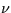Next: 12.8 Low-Dispersion Merged Extracted Up: 12 Final Archive Data Previous: 12.6 Low-Dispersion Resampled Image

12.7 High-Dispersion Resampled Image FITS File (SIHI)

The SIHI contains more information than stored in the corresponding low-dispersion file and, as a result, the FITS format is slightly more complex. Overall, the SIHI is comprised of a primary array containing the resampled image, a binary table of wavelengths and both predicted and found line positions, an image extension offlags, and a second image extension of background cosmic ray flags.

The high-dispersion SI data is similar to the low-dispersion SI data except that the high-dispersion wavelength linearization varies with spectral order, and the entire image is stored in the primary array. Each pixel is resampled to the position determined by the summation of the vectors computed for:

• shift to photometric correction (ITF) raw space,
• shift from ITF space to geometrically-rectified space,
• rotation such that orders are horizontal,
• wavelength linearization,
• adjustment to maintain the echelle orders at approximately the same locations in the file in the spatial direction,
• corrections for the spatial deviations (cross-dispersion wiggles) for LWP, LWR, and SWP data,
• heliocentric velocity correction, and
• de-splaying correction.
The high-dispersion SI is stored in the SIHI as a 2-D (768 samples × 768 lines) primary array. Each pixel represents an FN scaled up by a factor of 32 for storage purposes. The pixels are coded as 16-bit, two's complement integers, with the bits stored in decreasing order of significance. When the image is displayed with the origin in the lower left corner, the short-wavelength, closely-spaced high order numbers appear at the bottom, and the long-wavelength, low order numbers appear at the top. Within each order, the wavelengths increase from left to right.

Because the wavelength linearization varies with spectral order, the starting wavelength and wavelength increment values vary with each order. This information is stored in a binary table extension to the SIHI, which follows the primary array. The entire contents of the binary table extension include:

• Order Number, one 8-bit integer.
• Starting wavelength, one double-precision floating point number. Heliocentric velocity correction has been applied.
• Wavelength increment, one double-precision floating point number.
• predicted line position of order centroid, one single-precision floating point number.
• line position where spectral centroid is found, one single-precision floating point number. (This is determined by the high-dispersion spectral flux extraction module and written back into the SIHI file retroactively.)
The associatedflags and cosmic ray flags are stored in the SIHI image extensions with the same dimensions and orientation as the high-dispersion SI data contained in the primary array. The pixel quality flags are stored as unscaled 16-bit integers, and the cosmic ray flags are unscaled 8-bit integers. Table 12.9 shows the basic FITS keywords for the main and extension headers for the SIHI.

Table 12.9:  SIHI - Basic FITS Keywords
Keyword and value Description
 SIMPLE = T Standard FITS Format
 BITPIX = 16 16-bit 2's complement pixels
 NAXIS = 2 Two-dimensional image
 NAXIS1 = 768 Dimension along x-axis
 NAXIS2 = 768 Dimension along y-axis
 EXTEND = T Extensions are present
 CTYPE1 = 'SAMPLE '  x-axis
 CTYPE2 = 'LINE '  y-axis
 BUNIT = 'FN '  Flux Numbers
 BSCALE = 3.1250E-02 real=tape*bscale+bzero
 BZERO = 0. offset
 TELESCOP= 'IUE '  International Ultraviolet Explorer
 FILENAME= 'AAAnnnnn.SIHI'  Filename (camera)(number).SIHI
 DATE = 'dd/mm/yy'  Date file was written
 ORIGIN = 'VILSPA '  Institution generating the file
 DATAMIN = nnnnn.n  Minimum pixel value
 DATAMAX = nnnnn.n  Maximum pixel value
 XTENSION= 'BINTABLE'  Binary table extension
 BITPIX = 8 Binary data
 NAXIS = 2 Two-dimensional table array
 NAXIS1 = 25 Width of table in bytes
 NAXIS2 = nn Number of entries in table
 PCOUNT = 0 Number of bytes following data matrix
 GCOUNT = 1 Only one group
 TFIELDS = 5 Number of fields in each row
 TFORM1 = '1B '  8-bit byte
 TTYPE1 = 'ORDER '  Order number
 TUNIT1 = ' '  Unitless
 TFORM2 = '1D '  Double precision floating point
 TTYPE2 = 'WAVELENGTH'  Starting wavelength
 TUNIT2 = 'ANGSTROM'  Unit is angstroms
 TFORM3 = '1D '  Double precision floating point
 TTYPE3 = 'DELTAW '  3rd field is wavelength increment
 TUNIT3 = 'ANGSTROM'  Unit is angstrom
 TFORM4 = '1E '  Single precision floating point
 TTYPE4 = 'LINE_PREDICTED'  Predicted line position of order centroid
 TUNIT4 = 'PIXEL '  Unit is pixel
 TFORM5 = '1E '  Single precision floating point
 TTYPE5 = 'LINE_FOUND'  Line number where spectral centroid is found
 TUNIT5 = 'PIXEL '  Unit is pixel
 FILENAME= 'AAAnnnnn.WLHI'  Filename (camera)(number).WLHI
 EXTNAME = 'SIHIW '  Name of table
 XTENSION= 'IMAGE '  Image extension
 BITPIX = 16 16-bit 2's complement pixels
 NAXIS = 2 Two-dimensional image
 NAXIS1 = 768 Dimension of x-axis
 NAXIS2 = 768 Dimension of y-axis
 PCOUNT = 0 Number of bytes following data matrix
 GCOUNT = 1 Number of groups
 CTYPE1 = 'SAMPLE '  X-axis
 CTYPE2 = 'LINE '  Y-axis
 BUNIT = ' '  Unitless
 FILENAME= 'AAAnnnnn.SFHI'  Filename (camera)(number).SF(disp)
 EXTNAME = 'SIHIF '  SIHI pixel quality flags
 XTENSION= 'IMAGE '  Image extension
 BITPIX = 8 8-bit integer pixels
 NAXIS = 2 Two-dimensional image
 NAXIS1 = 768 Dimension of x-axis
 NAXIS2 = 768 Dimension of y-axis
 PCOUNT = 0 Number of bytes following data matrix
 GCOUNT = 1 Number of groups
 CTYPE1 = 'SAMPLE '  X-axis
 CTYPE2 = 'LINE '  Y-axis
 BUNIT = ' '  Unitless
 FILENAME= 'AAAnnnnn.CRHI'  Filename (camera)(number).CR(disp)
 EXTNAME = 'SIHIC '  SIHI cosmic ray background flagsNext: 12.8 Low-Dispersion Merged Extracted Up: 12 Final Archive Data Previous: 12.6 Low-Dispersion Resampled Image
Karen Levay
12/4/1997